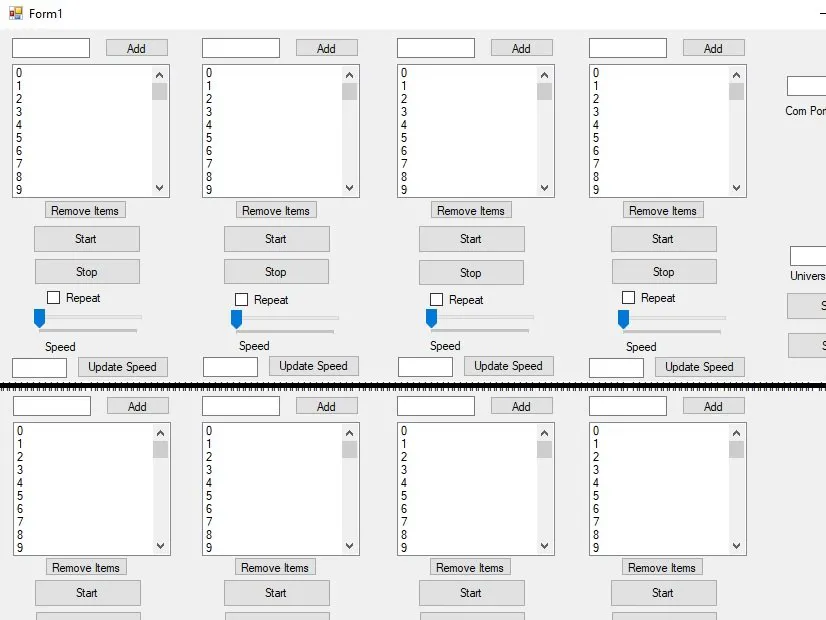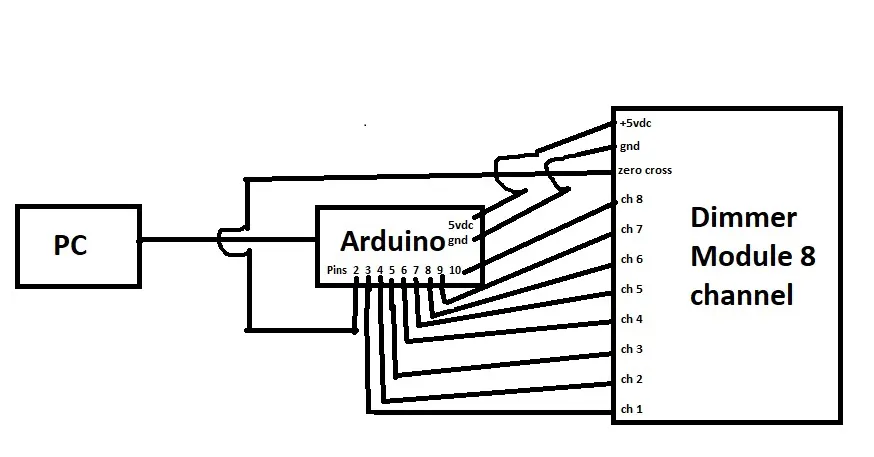Published

# Windows interface to have total control over lamps

Brightness control or simply turn on/ turn of. Everything done automatically creating beautiful effects with lamps. Cool for a mini party.

BeginnerWork in progress341## Things used in this project

### Hardware componentsArduino UNO We also need: - Dimmer ac 220v with zero cross detector compatible with Arduino ( 1, 2, 3, 4, 5, 6, 7 or 8 channel ) - Dimmable lamps
×1

## Schematics

### Diagram

Diagramproject

## Code

Arduino
```#include <TimerOne.h>                        // Avaiable from http://www.arduino.cc/playground/Code/Timer1

volatile int i, j;                        // Variable to use as a counter
volatile boolean zero_cross = {0,0,0,0,0,0,0,0};  // Boolean to store a "switch" to tell us if we have crossed zero
int AC = {3,4,5,6,7,8,9,10};                       // Setup the AC output pins
//int ACenable = {1,1,1,1,1,1,1,1};                 // Enable dimming for this output
long int output = {0,0,0,0,0,0,0,0};                 // Create output vars for Dimming level (0-128)  0 = on, 128 = 0ff
int dim = 0;                                 // Dimming level (0-128)  0 = on, 128 = 0ff
int freqStep = 78;                           // Set the delay for the frequency of power (65 for 60Hz, 78 for 50Hz) per step (using 128 steps)
volatile long periodSerial;                                            // freqStep may need some adjustment depending on your power the formula
int channel;                                 // you need to us is (500000/AC_freq)/NumSteps = freqStep
// You could also write a seperate function to determine the freq

void setup() {                                      // Begin setup
pinMode(AC, OUTPUT);                           // Set the Triac pin as output
pinMode(AC, OUTPUT);
pinMode(AC, OUTPUT);                           // Set the Triac pin as output
pinMode(AC, OUTPUT);
pinMode(AC, OUTPUT);                           // Set the Triac pin as output
pinMode(AC, OUTPUT);
pinMode(AC, OUTPUT);                           // Set the Triac pin as output
pinMode(AC, OUTPUT); // Set the Triac pin as output

attachInterrupt(0, zero_cross_detect, FALLING);   // Attach an Interupt to Pin 2 (interupt 0) for Zero Cross Detection
Timer1.initialize(freqStep);                      // Initialize TimerOne library for the freq we need
Timer1.attachInterrupt(output_check, freqStep);   // Use the TimerOne Library to attach an interrupt
// to the function we use to check to see if it is
// the right time to fire the triac.  This function
// will now run every freqStep in microseconds.
Serial.begin(9600);
}                                                   // End setup

void zero_cross_detect() {                 // function to be fired at the zero crossing
zero_cross = 1;                     // set the boolean to true to tell our dimming function that a zero cross has occured
zero_cross = 1;
zero_cross = 1;                     // set the boolean to true to tell our dimming function that a zero cross has occured
zero_cross = 1;
zero_cross = 1;                     // set the boolean to true to tell our dimming function that a zero cross has occured
zero_cross = 1;
zero_cross = 1;                     // set the boolean to true to tell our dimming function that a zero cross has occured
zero_cross = 1;
}                                          // End zero_cross_detect

void output_check() {                        // Function will fire the triac at the proper time

for( j=0; j<8; j++ ) {                     // Loop this function for each one of the outputs
if(zero_cross[j]) {                      // First check to make sure the zero-cross has happened else do nothing
if(i[j] >= output[j]) { // Check and see if i has accumilated to the dimming value we want
digitalWrite(AC[j], HIGH);           // Fire the Triac mid-phase
delayMicroseconds(8.33);                // Pause briefly to ensure the triac turned on
digitalWrite(AC[j], LOW);            // Turn off the Triac gate (Triac will not turn off until next zero cross)
i[j]=0;                              // If we fired the triac, reset the counters
zero_cross[j] = 0;                   // Reset the zero cross detection
} else {
i[j]++;                              // if nothing is done incriment th counter
}
}                                        // End zero_cross check
}                                          // End each loop
}                                            // End output_check function

void loop() {

while (!Serial) {}
channel = Serial.parseInt();
switch(channel){
case 1:
output=Serial.parseInt();
case 2:
output=Serial.parseInt();
case 3:
output=Serial.parseInt();
case 4:
output=Serial.parseInt();
case 5:
output=Serial.parseInt();
case 6:
output=Serial.parseInt();
case 7:
output=Serial.parseInt();
case 8:
output=Serial.parseInt();

}
}
```

## Credits

### mamífero

6 projects • 11 followers